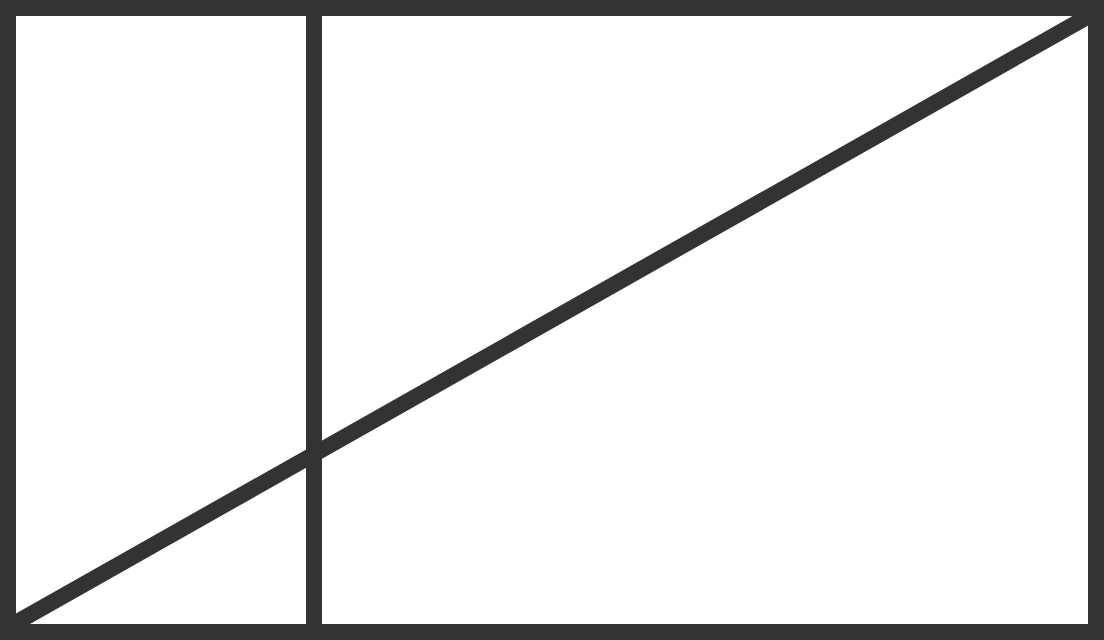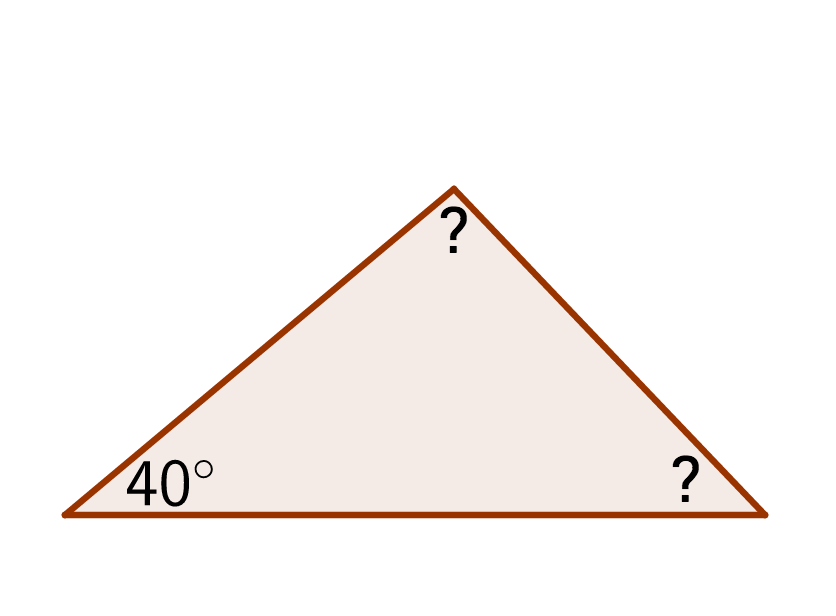Geometry

# Classification of Triangles Warmup

True or False?

A right triangle can be isosceles.Above, a line cuts across two adjacent rectangles.

How many right triangles can you find in this diagram?

Two sides of an isosceles triangle measure 3 and 5. What could be the length of the third side?Eli needs to draw an obtuse triangle and begins by creating a $40^\circ$ angle. What could be the measure of the next angle he draws? (In an obtuse triangle, one angle measures more than $90^\circ.$

What type of triangle has both an $89^\circ$ angle and a $1^\circ$ angle?

×